# IF-ELSE Excel Functions

What is IF-ELSE functions in Excel:
Definition: The IF function in excel returns one value if the condition is True and another value if it’s false
How IF functions  work: Arguments/Syntax: IF ([Condition],[Value1],[Value2])
[Condition]: It can be any logical condition like greater than, smaller than or equals to value
[Value1]: This value will get returned when the specified condition is true
[Value2]: This value will get returned when the specified condition is false
For the following different used cases of IF-ELSE functions consider the following data in excel: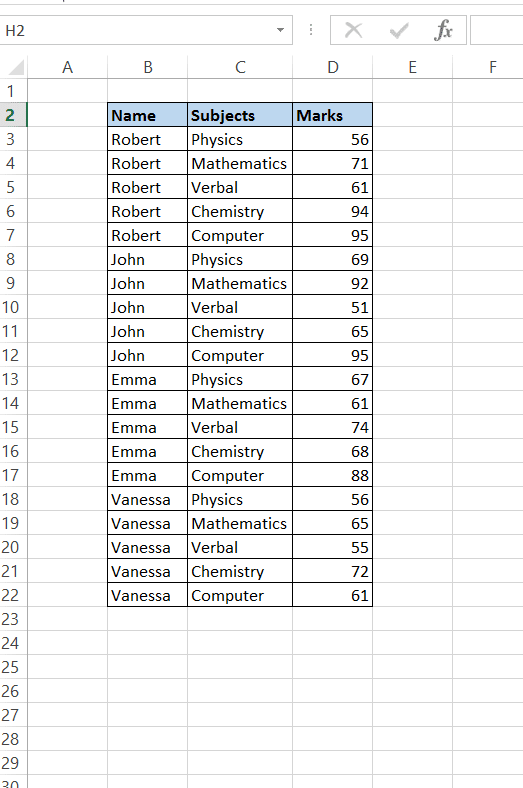## The  Basics

1. IF – ELSE Function:
Example: Consider the same dataset as given above. Now suppose based on the marks obtained by each person, if the marks is greater than 60 then the person is “Pass” in that subject else “Fail”. So, to assign “Pass or Fail’ we can use IF function: IF(D3>60,”Pass”,”Fail”)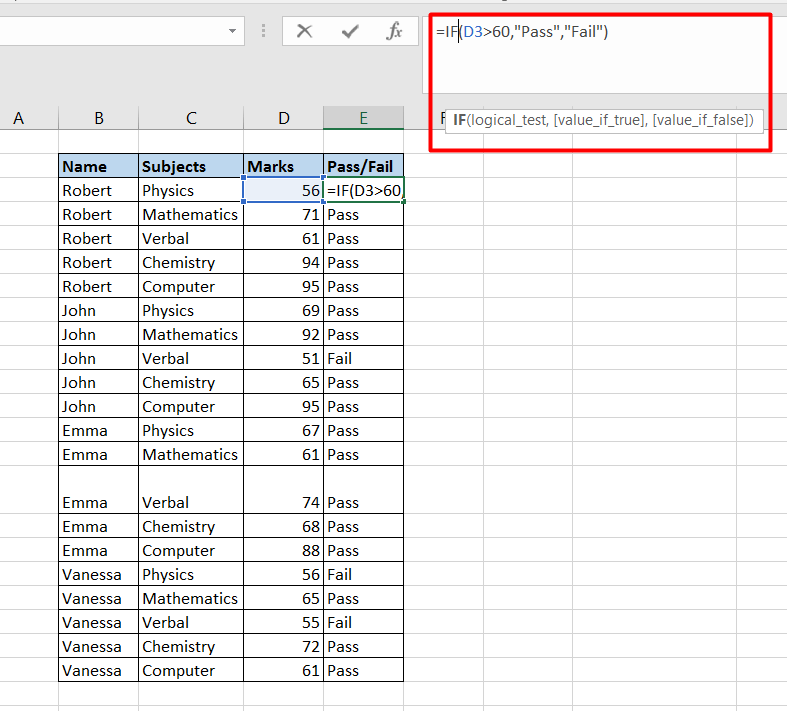## Using Multiple IF Statement in 1 Formula

2. Nested IF-ELSE: In nested IF-ELSE

When to use this: there are multiple if-else conditions mentioned in same formula.
Example: Consider the same dataset as given above. Now suppose the objective is to assign grades based on the marks obtained by each person. So, if marks are greater than 80 then its “A” grade, if marks is greater than 60 and less than 80 then its “B” grade otherwise “C” grade: IF(D3>=80, “A”, IF(D3>=60,”B”,”C”))
How nested If-Else works: First formula evaluates the condition of 1st IF’s statement, if the condition is true then it returns the “A” but if it is false then formula evaluates the condition of 2nd IF’s statement and now if 2nd IF’s statement condition is true then it will return “B” value otherwise “C”.3. Nested IF-ELSE with Conditional Operator Functions (AND/OR):
a) IF-ELSE with AND Function:
Example: Consider the same dataset as given above. Now suppose the objective is to segregate those names who are both “Pass” and have grade as “A” from those who are “Pass” but have grade as “B”. To do that we need to use both “If-Else” and “And functions”:
IF(AND(E3=”Pass”,F3=”A”),”5 cgpa”,IF(AND(E3=”Pass”,F3=”B”),”4 cgpa”,”3 cgpa”))
How it works: First formula evaluates the condition of 1st IF’s statement, now if both the conditions under “AND” statement are true then it returns the “5 cgpa” value but if any of the condition within “AND” is false then formula evaluates the “AND” condition present in 2nd IF’s statement and now again if both the conditions of within 2nd “AND” are true then then it will return “4 cgpa” value otherwise “3 cgpa”.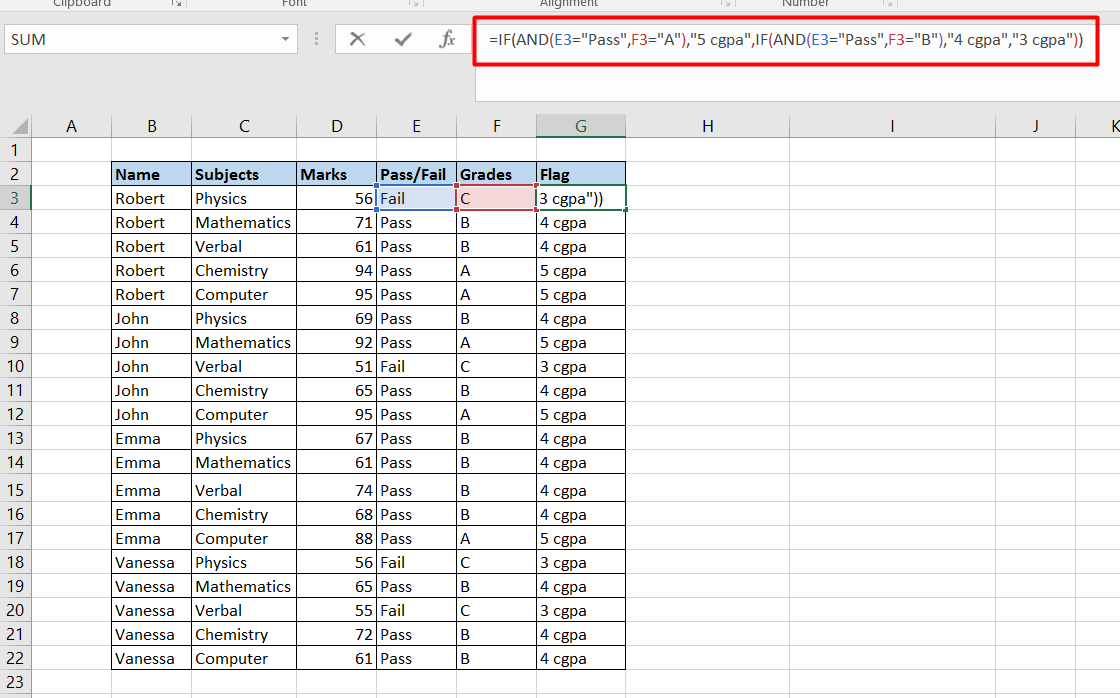b) IF-ELSE with OR Function: Consider the same example as above but now instead of using “AND” function, we will use “OR” function :
IF(OR(E3=”Pass”,F3=”A”),”5 cgpa”,IF(OR(E3=”Pass”,F3=”B”),”4 cgpa”,”3 cgpa”))
How it works: First formula evaluates the condition of 1st IF’s statement, now if any of the conditions under “OR” statement is true then it returns the “5 cgpa” value but if both of the condition within “OR” are false then formula evaluates the “OR” condition present in 2nd IF’s statement and now again if any of the conditions of within 2nd “OR” is true then then it will return “4 cgpa” value otherwise “3 cgpa”.## Averaging and Summing with IF Functions

4. Inbuilt IF function with Aggregation Functions
a) SUMIF:
Definition: The SUMIF function in excel is used to sum only those values in the given range that meet the criteria given by the user
Arguments:
Range: It is required argument. It takes array or range of cells that user wants to be evaluated by criteria.
Criteria: It is required argument. The criteria is in the form of a number, expression or a cell reference. For example, criteria can be expressed as 32, “>32”, B5, “32”, “apples”
Sum Range: It is an optional argument. This is required only when the user wants to add cells other than those specified in the Range argument
Example:
Consider the same data as shown above. Now, if instead of SUM of all the marks, if we require the SUM of marks of “Emma” alone then we use this SUMIF function:
SUMIF(\$B\$3:\$B\$22,G4,\$D\$3:\$D\$22)b) SUMIFS:
Definition: The SUMIFS function in excel is used to sum only those values in the given range that meet the multiple criteria given by the user
Arguments:
Sum Range: It is required argument. Array or range of cells to sum
Criteria Range1: It is required argument. It takes array or range of cells that user wants to be evaluated by criteria1.
Criteria1: It is required argument. The criteria that defines which cells in Criteria Range1 will be added. For example, criteria can be expressed as 32, “>32”, B5, “32”, “apples”
Criteria Range2: It is optional argument. It takes another array or range of cells that user wants to be evaluated by criteria2.
Criteria2: It is optional argument. The criteria that defines which cells in Criteria Range2 will be added. For example, criteria can be expressed as 32, “>32”, B5, “32”, “apples”
Example: Consider the same data as shown above. If we require the SUM of marks of only “Robert” greater than 75 we use this SUMIFS function: SUMIFS(\$D\$3:\$D\$22,\$B\$3:\$B\$22,”Robert”,\$D\$3:\$D\$22,”>75″)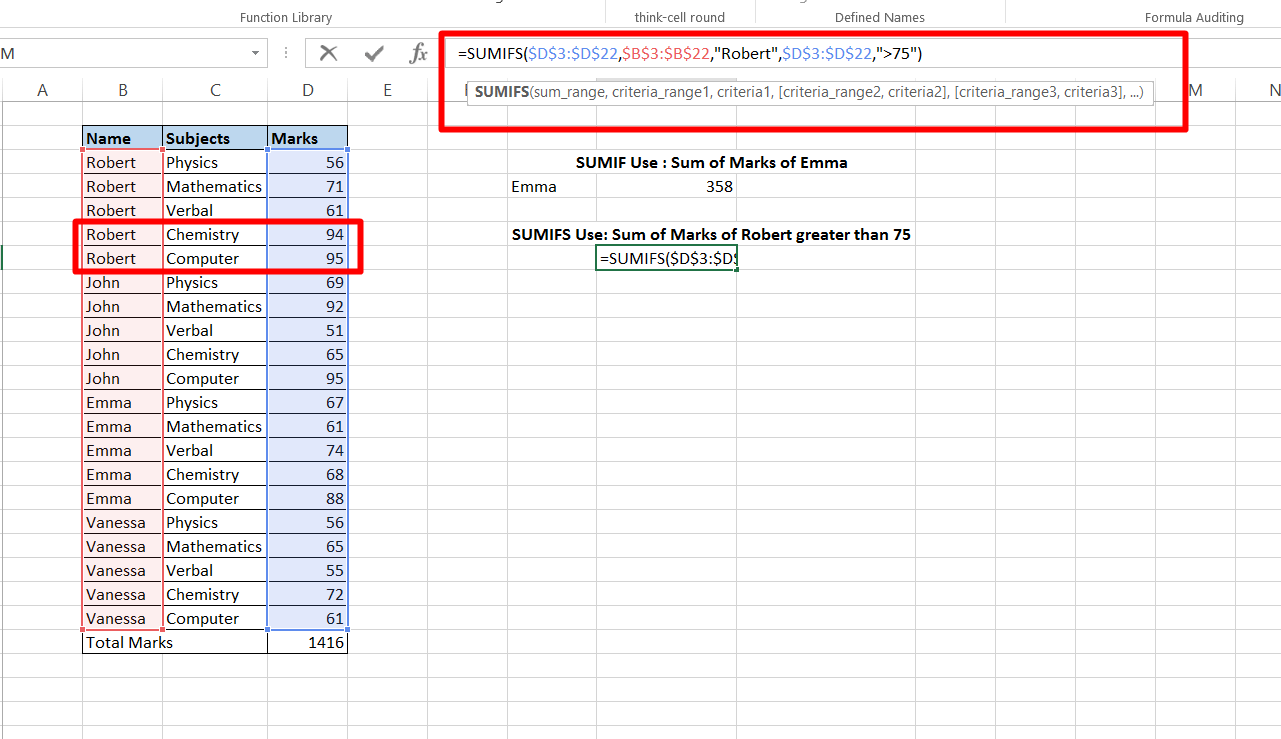c) COUNTIF:
Definition: The COUNTF function in excel is used to count only those cells that meet the criteria given by the user
Arguments:
Range: It is required argument. It takes array or range of cells that user wants to be evaluated by criteria.
Criteria: It is required argument. The criteria is in the form of a number, expression or a cell reference. For example, criteria can be expressed as 32, “>32”, B5, “32”, “apples”
Example:
Consider the same data as shown above. Now, if instead of total count of all the rows, if we require the number of rows which has “Emma” as name then we use this COUNTIF function: COUNTIF(\$B\$3:\$B\$22,”Emma”)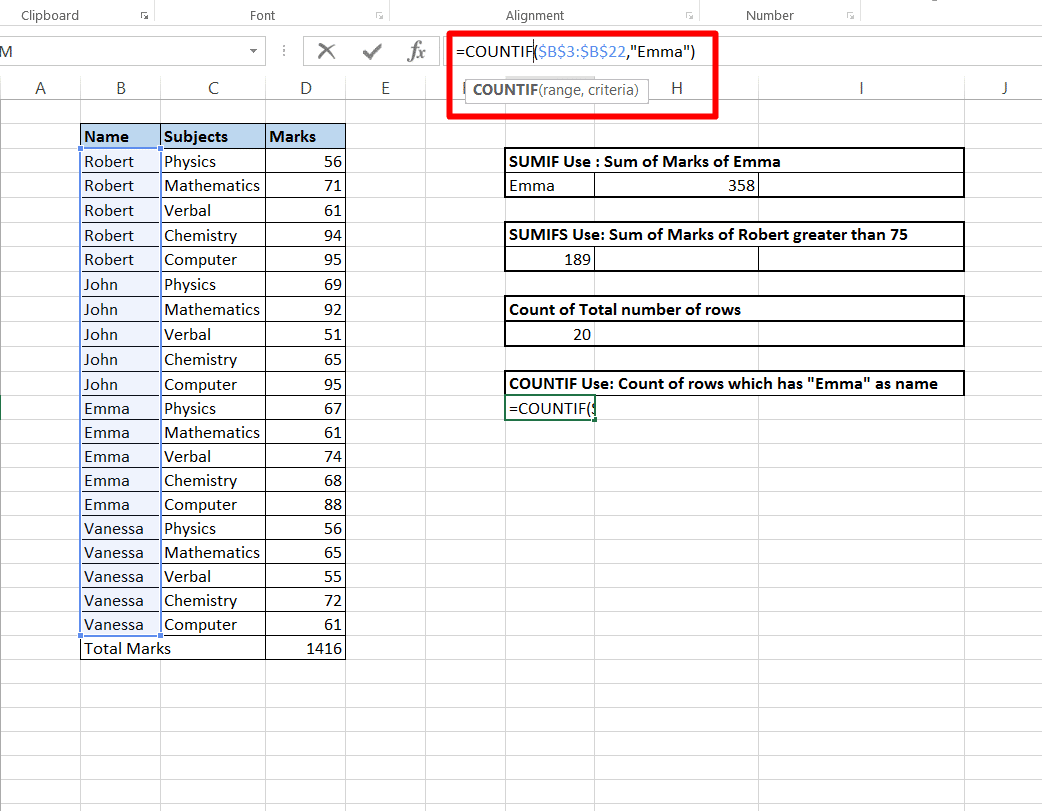d) COUNTIFS:
Definition: The COUNTIFS function in excel is used to count only those values in the given range that meet the multiple criteria given by the user
Arguments:
Criteria Range1: It is required argument. It takes array or range of cells that user wants to be evaluated by criteria1.
Criteria1: It is required argument. The criteria that defines which cells in Criteria Range1 will be counted. For example, criteria can be expressed as 32, “>32”, B5, “32”, “apples”
Criteria Range2: It is optional argument. It takes another array or range of cells that user wants to be evaluated by criteria2.
Criteria2: It is optional argument. The criteria that defines which cells in Criteria Range2 will be counted. For example, criteria can be expressed as 32, “>32”, B5, “32”, “apples”
Example: Consider the same data as shown above. If we require the count of rows which has “Robert” as name and marks greater than 75 then we use this COUNTIFS function: COUNTIFS(\$B\$3:\$B\$22,”Robert”,\$D\$3:\$D\$22,”>75″)e) AVERAGEIF:
Definition: The AVERAGEIF function in excel is used to take average of only those cells that meet the criteria given by the user
Arguments:
Range: It is required argument. It takes array or range of cells that user wants to be evaluated by criteria.
Criteria: It is required argument. The criteria is in the form of a number, expression or a cell reference. For example, criteria can be expressed as 32, “>32”, B5, “32”, “apples”
Average Range: It is an optional argument. This is required only when the user wants to take average of cells other than those specified in the Range argument
Example:
Consider the same data as shown above. Now, if instead of AVERAGE of all the marks, if we require the AVERAGE of marks of “John” alone then we use this AVERAGEIF function: AVERAGEIF(\$B\$3:\$B\$22,”John”,\$D\$3:\$D\$22)f) AVERAGEIFS:
Definition: The AVERAGEIFS function in excel is used to average only those values in the given range that meet the multiple criteria given by the user
Arguments:
Average Range: It is required argument. Array or range of cells to take average
Criteria Range1: It is required argument. It takes array or range of cells that user wants to be evaluated by criteria1.
Criteria1: It is required argument. The criteria that defines which cells in Criteria Range1 will be average. For example, criteria can be expressed as 32, “>32”, B5, “32”, “apples”
Criteria Range2: It is optional argument. It takes another array or range of cells that user wants to be evaluated by criteria2.
Criteria2: It is optional argument. The criteria that defines which cells in Criteria Range2 will be average. For example, criteria can be expressed as 32, “>32”, B5, “32”, “apples”
Example: Consider the same data as shown above. If we require the AVERAGE of marks of only “Vanessa” greater than 60 we use this AVERAGEIFS function: AVERAGEIFS(\$D\$3:\$D\$22,\$B\$3:\$B\$22,”Vanessa”,\$D\$3:\$D\$22,”>60″)70 Excel Keyboard Shortcuts